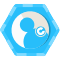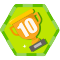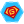## 求解表达式

\begin{bmatrix}
V_x\\
V_y \\
V_z
\end{bmatrix}=\begin{bmatrix}
\sum _i I_x(q_i)^{2} &\sum _i I_x(q_i) I_y(q_i) &\sum _i I_x(q_i) I_z(q_i) \\
\sum _i I_x(q_i) I_y(q_i) &\sum _i I_y(q_i)^{2} &\sum _i I_y(q_i) I_z(q_i) \\
\sum _i I_x(q_i) I_z(q_i)& \sum _i I_y(q_i) I_z(q_i) &\sum _i I_z(q_i)^{2}
\end{bmatrix}\begin{bmatrix}
-\sum_i I_xI_t(q_i)\\
-\sum_i I_yI_t(q_i)\\
-\sum_i I_zI_t(q_i)
\end{bmatrix}


## 高斯分布公式

f(x|\mu ,\sigma^{2} )=\frac{1}{\sqrt{2\pi \sigma^{2}}}e^{-\frac{(x-\mu^{2} )}{2\sigma^{2}}}


μ 是分布的平均值或期望值 （以及其中位数和模式 ）。

void cvCalcOpticalFlowPyrLK(
const CvArr* imgA,//初始图像
const CvArr* imgB,//最终图像
CvArr* pyrA,
CvArr* pyrB,//pyrA,B申请存放两幅图像金字塔的缓存
CvPoint2D32f* featuresA,//存放的是用于寻找运动的点
CvPoint2D32f* featuresB,//存放featuresA中点的新位置
int count,//featuresA中点的数目
CvSize winsize,//定义了计算局部连续运动的窗口尺寸
int level,//用于设置构建的图像金字塔的栈的层数，若设置为0，则不使用金字塔
char* status,//函数调用结束时，status中的每个元素被置1（对应点在第二幅图中发现）或者0（未发现）
float* track_error,//为可选参数，表示被跟踪点的原始图像小区域与此点在第二幅图像的小区域间的差的数组
CvTermCriteria criteria,//迭代终止条件
int flags//标志位
);


LK.cpp

#include<opencv2/opencv.hpp>
#include<iostream>
using namespace std;
const int MAX_CORNERS = 500;

int main()
{

CvSize img_sz = cvGetSize(imgA);
int win_size = 10;

IplImage *eig_image = cvCreateImage(img_sz, IPL_DEPTH_32F,1);
IplImage *tmp_image = cvCreateImage(img_sz, IPL_DEPTH_32F, 1);

int corner_count = MAX_CORNERS;
CvPoint2D32f *cornersA = new CvPoint2D32f[MAX_CORNERS];

cvGoodFeaturesToTrack(
imgA,
eig_image,
tmp_image,
cornersA,
&corner_count,
0.01,
5.0,
0,
3,
0,
0.04
);

cvFindCornerSubPix(
imgA,
cornersA,
corner_count,
cvSize(win_size, win_size),
cvSize(-1, -1),
cvTermCriteria(CV_TERMCRIT_ITER | CV_TERMCRIT_EPS, 20, 0.03)
);

char features_found[MAX_CORNERS];
float features_errors[MAX_CORNERS];
CvSize pyr_sz = cvSize(imgA->width + 8, imgB->height / 3);
IplImage *pyrA = cvCreateImage(pyr_sz, IPL_DEPTH_32F, 1);
IplImage *pyrB = cvCreateImage(pyr_sz, IPL_DEPTH_32F, 1);
CvPoint2D32f *cornersB = new CvPoint2D32f[MAX_CORNERS];

cvCalcOpticalFlowPyrLK(
imgA,
imgB,
pyrA,
pyrB,
cornersA,
cornersB,
corner_count,
cvSize(win_size, win_size),
5,
features_found,
features_errors,
cvTermCriteria(CV_TERMCRIT_ITER | CV_TERMCRIT_EPS, 20, 0.3),
0
);

for (int i = 0; i < corner_count; ++i)
{
if (features_found[i] == 0 || features_errors[i] > 550)
{
cout << "Error is " << features_errors[i];
continue;
}

CvPoint p0 = cvPoint(cvRound(cornersA[i].x), cvRound(cornersA[i].y));
CvPoint p1 = cvPoint(cvRound(cornersB[i].x), cvRound(cornersB[i].y));
cvLine(imgC, p0, p1, CV_RGB(255, 0, 0),2);
}

cvNamedWindow("ImageA", 0);
cvNamedWindow("ImageB", 0);
cvNamedWindow("LKpyr_OpticalFlow", 0);
cvShowImage("ImageA", imgA);
cvShowImage("ImageB", imgB);
cvShowImage("LKpyr_OpticalFlow", imgC);
cvWaitKey(0);
return 0;
}



## 文尾### Pulsar-V### 评论(0)

Optical Flow介绍与代码实现

Optical Flow介绍与代码实现 介绍 首先我们先来介绍一下Optical Flow是个什么东西, 在浏览器的搜索框框里面我们输入"Optical flow"可以看到维基百科的解释: 光流(Optical flow or optic flow...

2018/09/18
0
0

AI科技大本营
2018/11/22
0
0

2018/05/19
9.3K
0
【联想】使用opencv接口进行人脸识别算法优化

2018/06/04
0
0

0 写在前面 这篇博客主要参考资料为《OpenCV 3计算机视觉Python语言实现》（Learning OpenCV 3 Computer Vison with Python）。 因为之前用Faster R-CNN做过一个红绿灯检测的小实践，但是Git...

learning_tortosie
2018/04/12
0
0

18280800244
13分钟前
20
0

18281961458
14分钟前
39
0

18281771440
14分钟前
57
0

18249436294
15分钟前
29
0

18249436304
15分钟前
39
0